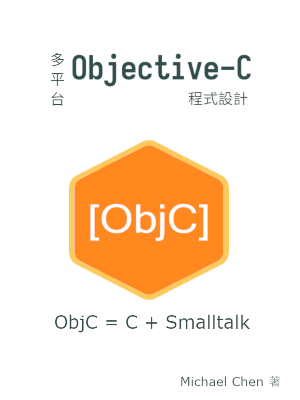# 撰寫 Objective-C 的類別 (Class) 和物件 (Object)

## 前言

除了使用在 Cocoa 或 GNUstep 中已存在的類別外，我們也可以利用 Objective-C 的物件系統建立新的類別。由於 Objective-C 是 C 的延伸，實作類別時仍然會用到 C 的部分來寫一些指令，而類別件相關的語法則由 Objective-C 所提供。本文以簡單的範例來看如何在 Objective-C 中建立類別。

## Objective-C 程式碼的架構

除了主程式外，大部分 Objective-C 程式會以物件 (object) 的形式來運行。物件根據類別 (class) 來生成。撰寫類別是撰寫 Objective-C 程式的基礎工作。

撰寫 Objective-C 類別時，類別的公開界面和內部實作會拆開在兩個檔案。公開界面位於標頭檔中，內部實作放在原始檔中。使用標頭格和原始碼是相容於 C 的設計。

## 宣告類別的公開界面

Objective-C 的公開界面的虛擬碼如下：

``````@interface Klass : Base {
/* Instance variable declarations. */
}

/* Public message declarations. */
@end
``````

`@interface``@end` 是固定的語法，公開界面寫在兩者所包起來的區塊中。

Klass 代表此類別的名稱，而 Base 代表所繼承的類別名稱。典型的 Objective-C 類別至少會繼承 `NSObject` 這個共通的基礎類別，完全不繼承任何類別的類別甚少。

在一對大括號 `{``}` 所包住的區塊是實體變數 (instance variable) 的宣告區塊。其他的部分則是訊息 (message) 的宣告區塊。Objective-C 使用訊息而非主流物件導向程式中的方法 (method) 是因為兩種物件系統在運行期的行為上有差異。

Objective-C 的訊息可細分為實體訊息 (instance message) 和類別訊息 (class message)。兩者的差別在傳入的物件是實體 (instace) 還是類別 (class)。另外，類別本身視為特殊物件，所以兩者語法雷同，這是承襲自 Smalltalk 的特性。

實體訊息的虛擬碼如下：

``````-(type) instanceMessageWith: (type_a)a andObj: (type_b b;
``````

訊息 `instanceMessageWith:andObj:` 前綴使用減號 `-` 代表該訊息是實體訊息。本範例訊息接收兩個參數，參數的型態分別是 `type_a``type_b`。該訊息回傳的型態為 `type`

類別訊息的虛擬碼如下：

``````+(type) classMessageWith: (type_a)a;
``````

訊息 `classMessageWith:` 前綴使用加號 `+` 代表該訊息是類別訊息。本範例訊息接收一個參數，該參數型態為 `type_a`。該訊息的回傳型態為 `type`

## 宣告座標點類別 `Point` 的公開界面

接下來，我們用實際的範例來展示其寫法。在本文中，我們使用平面座標點 (point) 當成範例類別。採用這個例子的原因是點易於實作，可以專心在練習類別和物件的語法上。

以下是 `Point` 類別的公開界面：

``````/* point.h */
#pragma once

#import <Foundation/Foundation.h>

@interface Point : NSObject {
double x;
double y;
}

+(Point *) new;
+(Point *) newWithX: (double)px andY: (double)py;

+(double) distanceBetween: (Point *)p andPoint: (Point *)q;

-(Point *) init;
-(Point *) initWithX: (double) px andY: (double)py;

-(double) x;
-(double) y;
@end
``````

我們先來看屬性的部分：

``````@interface Point : NSObject {
double x;
double y;
}
``````

這個部分告訴編譯器我們的類別是 `Point`，有兩個屬性 `x``y`。此 `Point` 屬性繼承 `NSObject` 類別。Objective-C 採用單一基礎類別，大部分的類別至少會繼承 `NSObject` 類別，若有需要也可以繼承其他的類別。

Objective-C 沒有真正為建構子 (constructor) 而設的語法，使用一般的訊息充當建構子即可。本範例程式宣告了四個建構訊息：

``````+(Point *) new;
+(Point *) newWithX: (double)px andY: (double)py;

-(Point *) init;
-(Point *) initWithX: (double) px Y: (double) py;
``````

前兩者是類別訊息，後兩者是實體訊息。使用兩套訊息的意義在於讓類別使用者依照自己的習慣來宣告變數。使用不帶參數的 `new``init` 時會自動將點設置在 `(0.0, 0.0)`

雖然我們可以用任何名字來當成建構子訊息，一般常用的訊息名稱是 `init``new`。兩者的差別在於 `init` 訊息多不配置記憶體，只負責初始化物件，而 `new` 則會一併配置記憶體和初始化物件。但這不是強制性的，只是習慣，所以還是得閱讀該類別的 API 手冊才知道該訊息實際的行為。

注意一下我們的建構子訊息是實體訊息，而非類別訊息，這似乎和主流程式語言略為不同。因為 Objective-C 建立物件的敘述如下：

``````Point *p = [[Point alloc] init];
``````

在這行敘述中，`[Point alloc]` 會為 `Point` 物件配置記憶體，但這時候該物件還未初始化。接著，對配置好的匿名物件傳入 `init` 訊息，完成初始化的動作。

使用 `new` 訊息時，建立物件的敘述會變成：

``````Point *p = [Point new];
``````

因為 `new` 在內部偷偷地進行 `alloc``init` 兩個訊息的動作，所以我們只要寫單一訊息即可。

我們另外用一個類別訊息來計算兩點間的距離：

``````+(double) distanceBetween: (Point *)p andPoint: (Point *)q;
``````

該訊息的使用方式如下：

``````/* p and q are instances of `Point`. */
double dist = [Point distanceBetween: p andPoint: q];
``````

類別訊息和實體訊息主要的差別是訊息所傳遞的物件相異，在語法上則雷同。以本範例來說，`distanceBetween: andPoint:` 是類別訊息，故會傳遞給 `Point` 類別。

其實這裡不一定非得用類別訊息來寫，也可以用實體訊息來寫。Objective-C 在這方面相當自由。

## 實作類別的內部

實作部分的虛擬碼如下：

``````@implementation Klass
+(type_2) classMessageWith: (type_a)a
{
/* Implement class message here. */
}

-(type_1) instanceMessageWith: (type_a)a andObj: (type_b)b
{
/* Implement instance message here. */
}
@end
``````

Objective-C 的類別實作放在一對 `@implementation``@end` 所包起來的區塊。 Klass 是該類別的名稱。每個訊息再分別以一對大括號 `{``}` 區隔開來。在公開界面中宣告的訊息，在原始碼中都得有相對應的實作。

## 實作座標點類別 `Point` 的內部

接著，我們分段來看 `Point` 類別的內部實作。先來看建構訊息：

``````+(Point *) newWithX: (double) px andY: (double) py
{
return [[[self class] alloc] initWith: px andY: py];
}
``````

由此可知，類別建構訊息只是在內部自動配置物件後再呼叫實體建構訊息而已。接著來看實體建構訊息：

``````-(Point *) initWithX: (double) px andY: (double) py
{
if (!self)
return self;

self = [super init];

x = px;
y = py;

return self;
}
``````

為什麼一開始會有這段程式碼呢？

``````if (!self)
return self;
``````

這是因為 Objective-C 採用兩段訊息來建立物件：

``````Point *p = [[Point alloc] init];
``````

`[Point alloc]` 訊息完成後，會配置一個尚未初始化的物件，該物件在建構子訊息中相當於 `self`，所以我們要檢查 `self` 本身不為空。

接著，我們先用父類別的初始化訊息來初始化 `self` 物件：

``````self = [super init];
``````

這是因為 `Point` 類別繼承自 `NSObject` 類別，所以要先由父類別初始化後，再由本類別繼續完成初始化的動作。

以本範例程式來說，我們只要將 `x``y` 的座標值存入物件即可。雖然 Objectiive-C 有 `self` 指標，但沒有 `self->x` 這種寫法，所以在命令參數時，要用相異的名稱，像是這裡的 `x``px`，才能區分內部變數和外部參數。

接著，我們接著來看求兩點距離的訊息：

``````+(double) distanceBetween: (Point *)p andPoint: (Point *)q
{
double dx = [p x] - [q x];
double dy = [p y] - [q y];
return sqrt(pow(dx, 2) + pow(dy, 2));
}
``````

注意到我們在這裡呼叫自己所實作的其他訊息。由於訊息在本質上是一種特化的函式，所以可在訊息實作區塊內呼叫其他訊息。

另外，我們在求距離時，仍然會用到 C 語言的 `pow()` 函式及 `sqrt()` 函式。如同我們前文所述，學 C 是學 Objective-C 的預備知識，因為我們還是有機會用 C 來處理實作類別的細節。

我們在本節末段列出 `Point` 類別的範例實作，給讀者參考：

``````/* point.m */
#include <math.h>
#import "point.h"

@implementation Point
+(Point *) new
{
return [[self class] newWithX: 0.0 andY: 0.0];
}

+(Point *) newWithX: (double) px andY: (double) py
{
return [[[self class] alloc] initWithX: px andY: py];
}

-(Point *) init
{
return [self initWithX: 0.0 andY: 0.0];
}

-(Point *) initWithX: (double)px andY: (double)py
{
if (!self)
return self;

self = [super init];

x = px;
y = py;

return self;
}

+(double) distanceBetween: (Point *) p andPoint: (Point *) q
{
double dx = [p x] - [q x];
double dy = [p y] - [q y];

return sqrt(pow(dx, 2) + pow(dy, 2));
}

-(double) x
{
return x;
}

-(double) y
{
return y;
}
@end
``````

## 使用 Objective-C 物件的外部程式

最後，我們以簡短的外部程式來看如何使用 `Point` 類別：

``````/* main.m */
#include <stdio.h>
#import "point.h"

int main(void)
{
NSAutoreleasePool* pool = [[NSAutoreleasePool alloc] init];
if (!pool)
return 1;

Point *p = nil;
Point *q = nil;

p = [[Point new] autorelease];
if (!p) {
perror("Failed to allocate p\n");
goto ERROR;
}

q = [[Point newWithX: 3.0 andY: 4.0] autorelease];
if (!q) {
perror("Failed to allocate q\n");
goto ERROR;
}

if (!(5.0 == [Point distanceBetween: p andPoint: q])) {
perror("Wrong distance\n");
goto ERROR;
}

[pool release];

return 0;

ERROR:
[pool release];

return 1;
}
``````

在這個範例中，我們用兩種訊息分別建立物件 `p` 和物件 `q`，再求其距離。最後釋放掉所配置的記憶體。程式中已經包含錯誤處理的部分。

細心的讀者可能會注意到我們並沒有為 `Point` 類別實作 `alloc``release` 訊息，為什麼還是可以使用呢？因為 `Point` 類別繼承自 `NSObject` 類別，而 `NSObject` 類別已經幫我們實作了物件的基本訊息。

## 編譯範例程式

可參考以下指令來編譯範例程式：

``````\$ gcc -o point point.m main.m -lm -lobjc -lgnustep-base -I /usr/include/GNUstep -L /usr/lib/GNUstep
``````

這個編譯指令比較長是因為 Objective-C 不是類 Unix 系統的標準語言，GNUstep 也不是類 Unix 系統的標準物件庫，所以要自己指定 GNUstep 所在的路徑。目前的解決方式是改用我們先前提到的 GNUstep Make 來管理 Objective-C 專案。

## 結語

雖然本文所使用的範例相當簡單，但簡單的範例更可以突顯類別和物件相關的語法，不會被實作細節所困住。由於 Objective-C 中和物件相關的語法和主流語言差異較大，建議多讀一些範例或是自己動手寫一些小範例，慢慢體會 Objective-C 的物件系統。

## 電子書籍

如果你覺得這篇 Objective-C 的技術文章對你有幫助，可以看看這本 Objective-C 程式設計電子書：【分享本文】